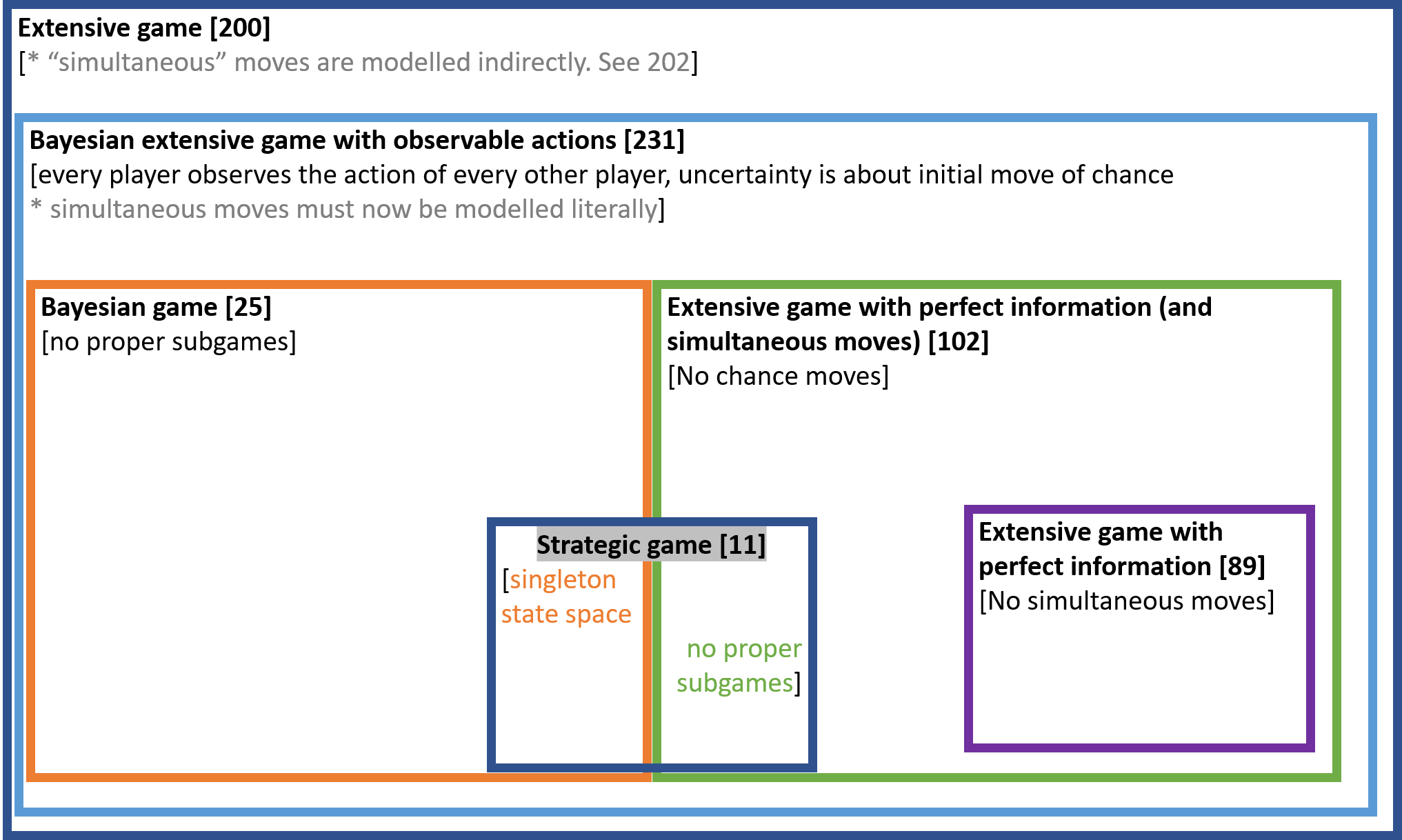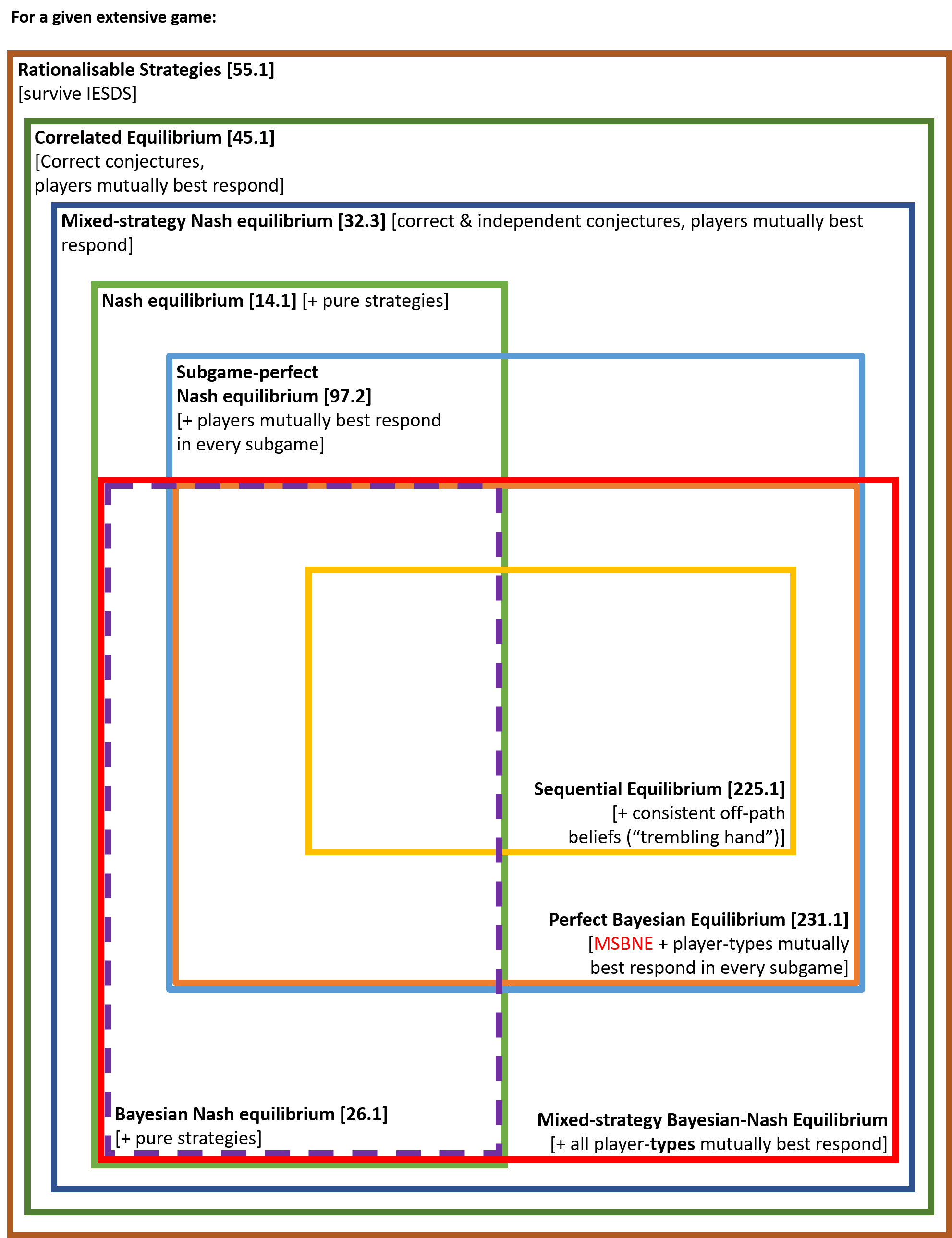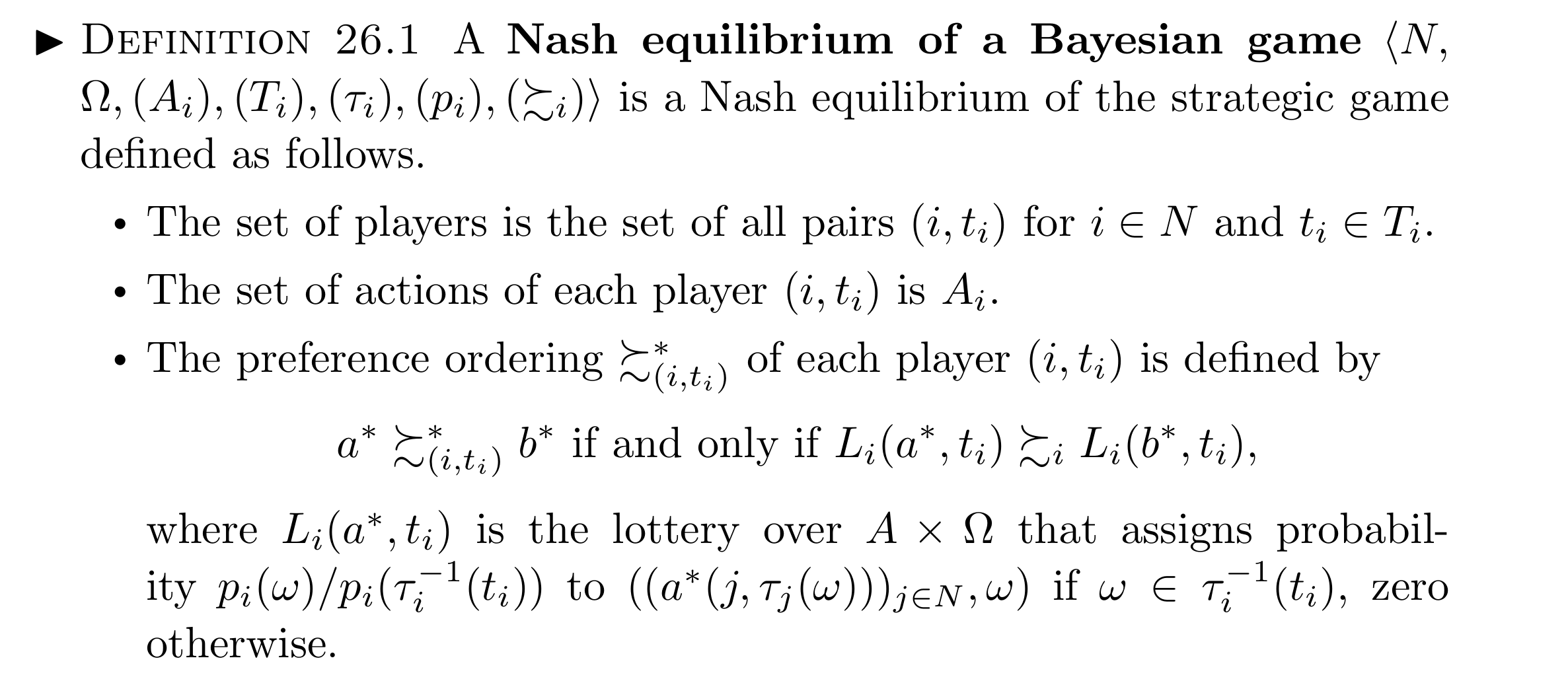# Should we prioritise cash transfers to the urban poor?

Note: I owe these ideas to Rossa O’Keeffe-O’Donovan and Natalie Quinn. However, the views expressed here are solely mine.

It’s well known that cash transfers have effects on prices. For instance, Cunha, De Giorgi and Jayachandran (2017) write:

[According to standard models], cash transfers increase the demand for normal goods, which will lead to price increases. This prediction holds either with perfect competition and marginal costs that are increasing in quantity, or with imperfect competition even if marginal costs are constant or decreasing […].

It’s helpful to think in terms of real goods instead of thinking in terms of money. Suppose there was a village in complete autarky: trade only occurs within the village. Say we gave everyone in the village $1,000. Nothing on the ground directly changes: they don’t get better tools, or more fertile land, just because we give them fiat money. What happens to consumption depends on the income distribution prior to the transfer. If all villagers have the same income, they will keep producing the same goods as before, and exchanging them in the same way, the prices will simply be higher1. If they had different incomes, the cash transfer will make them more equal in purchasing power, while also increasing prices (imagine if the transfer was a billion dollars). This amounts to a modest transfer of real goods from richer to poorer villagers2. Now suppose we only gave$1,000 to a randomly selected half of the villagers. Nothing immediately happens to the productive capacity of the village. Prices rise, which means purchasing power is transferred from non-recipients to recipients. The exact effect on consumption is a bit complicated. In an extremely simple economy where everyone consumes as much as possible of the staple good, recipients consume more, non-recipients consume less, and that’s the end of it. More realistically, the recipients create some new demand for luxury goods. Regardless, the welfare effect of the purchasing power transfer seem likely to be negative. What certainly doesn’t happen is that real goods are transferred from the person who funded the cash transfer programme (say, a GiveDirectly donor) to anyone in the village. This is impossible since the village is in autarky.

# Even imperceptible price effects can change everything

If the village were trading with other villages, the price increases would be more spread out, and the effect would be a transfer of purchasing power to the recipients from everyone else in the trade market. More generally, a cash transfer to recipient A is a real good transfer to A, from everyone who trades with A, directly or indirectly. An interesting empirical point is the following. If the market is large, the price effects could be spread across tens of thousands of people, thus they would be very small for each person. Then extremely high statistical power is required to distinguish these price effects from zero. But imperceptible effects on large numbers of people can still be very morally important (see Parfit’s Five Mistakes in Moral Mathematics). In this case, the imperceptible price effects reflect the fact that the cash transfer can’t increase real consumption within the market. So they are everything that matters!

In the case of GiveDirectly, things aren’t quite as bad as in my toy examples above. GiveDirectly targets the poorest inhabitants in a village, so even if the village were in complete autarky, GiveDirectly would cause a real goods transfer from richer inhabitants of the village to the poorer inhabitants (and a much bigger one than if the transfer was given to everyone). If all of western Kenya was in autarky, but there was costless trading within, the transfer would be from everyone in western Kenya (weighted by how much they consume) to the recipients. Notice how different this is from what we might have expected before taking into account price effects: a real transfer from the GiveDirectly donor to the recipient.

Cunha and colleagues’ empirical findings corroborate this:

In the more economically developed villages in the sample, households’ purchasing power is only modestly affected by these price effects. In the less developed villages, the price effects are much larger in magnitude, which we show is partly due to these villages being less tied to the outside economy and partly due to their having less competition among local suppliers.

Now let’s take another case: you give money to your neighbour (who like you, has perfect access to the world economy). There is no price effect. You both would have spent the money on the world market. There is a real goods transfer from you to your neighbour. This is the only case where reality perfectly matches intuition. The same would happen if your neighbour were a Kenyan who has access to world markets3.

# The upshot

Now, to me there is an obvious policy implication here: you should transfer cash not to the poorest, but to those who score highest on some combination of low income and access to the world economy. Income being equal, you should transfer to the most globalised recipients you can find. The importance of this can be enormous. If you give to someone in an autarkic village, you transfer goods from poor Kenyans to very poor Kenyans. If you give to a globalised city-dweller, the real transfer is from yourself to the recipient.

I haven’t been able to find any discussion of this policy implication in the literature. This is quite surprising for something that naturally follows from supply and demand theory.

There could be practical problems with this policy. Most obviously, people who live in globalised cities are typically rich, and those who live in isolated villages are typically poor4. So the ideal recipient, say someone who lives in Mumbai but is as poor as a rural day labourer, may not exist. (It could also be harder to identify the poor in the cities. But the operation costs of a cash transfer programme could perhaps be lower.)

1. This is known as the classical dichotomy in economics.

2. The more equal village might also shift its production and consumption towards more staples and fewer luxuries.

3. Except for the fact that Kenya uses a different currency, so you have to take into account exchange rates, which might not be free-floating. I don’t understand macroeconomics, so let’s ignore that.

4. There is some empirical data on this, for example from the World Bank report Geographic Dimensions of Well-Being in Kenya: Where are the Poor? (henceforth GdWBK). GdWBK chapter 2 tells us that the “poverty line is determined based on the expenditure required to purchase a food basket that allows minimum nutritional requirements to be met (set at 2,250 calories per adult equivalent (AE) per day) in addition to the costs of meeting basic non-food needs”. The rural poverty line (Ksh 1,239) is less than half the urban poverty line (Ksh 2,648). I find it surprising that basic goods really are twice as expensive in urban areas, but let’s take these estimates at face value. GdWBK doesn’t directly give information about the income distribution, but it tells us about the poverty gap index in different areas.

Wikipedia defines the poverty gap index as $$\frac{1}{N} \sum_{j=1}^{q} \left( \frac{z-y_j}{z} \right)$$ where $$N$$ is the total population, $$q$$ is the total population of poor who are living at or below the poverty threshold, $$z$$ is the poverty line, and $$y$$ is the income of the poor individual $$j$$. In this calculation, individuals whose income is above the poverty line have a gap of zero. Multiplying the poverty gap index by $$\frac{N}{q}$$, we get $$PG_p = \frac{1}{q} \sum_{j=1}^{q} \left( \frac{z-y_j}{z} \right)$$, the average poverty gap among the poor.

From GdWBK chapter 5, I compute $$PG_p=49\%$$ for the rural areas of the Western District of Kenya, $$PG_p=33\%$$ for the district of Nairobi, implying that the Nairobian poor live on an average of 67% of the urban poverty line and the poor in rural parts of the Western District live on an average of 51% of the rural poverty line.

June 18, 2018

# Meta-ethical theories

Here’s a diagram of meta-ethical theories, loosely based on Figure 1.9 in Miller’s Introduction to contemporary metaethics.1

1. The javascript embedding might break one day. Backups are in .png and .xml

June 17, 2018

# Relationships between games and solution concepts

The basic notion of strategic game can be extended in two directions: adding imperfect information, and adding sequential moves. This gives nice matryoshka dolls of types of games, depicted below with page numbers in Osborne and Rubinstein (OR) in brackets.Each type of game has a solution concept that is arguably natural to it.

Game Natural solution concept
Extensive game Sequential equilibrium [225.1]
Bayesian extensive game with observable actions Perfect Bayesian equilibrium [231.1]
Extensive game with perfect information (and simultaneous moves) Subgame perfect equilibrium [97.2]
Bayesian Game Bayesian-Nash equilibrium [26.1]
Strategic game Mixed-strategy Nash equilibrium [32.3] or Nash equilibrium [14.1]

Applying the solution concept of a more general game to a more specific game can always be done, but it is uninteresting. For example, all Nash equilibria of a strategic game are trivially subgame perfect, and trivially Bayesian.

We can also apply the solution concept of a more specific game to a more general game, for example, we can find the Nash equilibria of a game with sequential moves; some of these will not be subgame perfect. Suppose we wanted to do the same thing with Bayesian games. I found that this does not work straight out of the box when using the definitions from OR and other standard textbooks. We will need to make some small changes. In 26.1, OR make the following definition1:

A Nash equilibrium of a Bayesian game with vNM preferences $$\langle N, \Omega,(A_i), (T_i), (s_i), (p_i), (u_i) \rangle$$ is a Nash Equilibrium of the strategic game with vNM preferences defined as follows:

• The set of players is the set of all pairs $$(i,t_i)$$ for $$i \in N$$ and $$t_i \in T_i$$

• For each player $$(i,t_i)$$, the set of actions is $$A_i$$

• For each player $$(i,t_i)$$, the preference function assigns to action $$a_i$$ the payoff

$\sum_{\omega \in \Omega} p_i(\omega \mid t_i )u_i [(a_i,\hat{a}_{-i}(\omega)), \omega ]$

where $$\hat{a}_{-i}(\omega)$$ is the profile of actions taken by every player $$(j,s_j(\omega)), j \neq i$$ in state $$\omega$$.

For the purpose of seeing the relationships between solution concepts, calling this a “Nash equilibrium” is unfortunate. When we introduce extensive games, we give a new name, “subgame perfect equilibrium” to its natural solution concept. So why re-use the name “Nash equilibrium” for the solution concept of Bayesian games? It would be preferable to call 26.1 a Bayesian-Nash equilibrium of a Bayesian game, as distinct from a Nash equilibrium of a Bayesian game in which the players do not condition on their type. That is, in what I propose to call a Nash equilibrium of a Bayesian game, players choose an unconditional strategy, and their utilities correspond to the expected utilities using their prior over types. Picture the players before they receive their signals. They have some prior over the signals they and their opponents will receive. They can average over this uncertainty and decide on a strategy. If these unconditional strategies are best responses to each other, we have a Nash equilibrium. On this definition, a Nash equilibrium of a Bayesian game ignores all the properly Bayesian features of the game, just like a Nash equilibrium of a sequential game ignores all the sequential information. Why wouldn’t you condition on your type? You would if you were rational! But if you were rational you would also never play a non-subgame perfect Nash equilibrium strategy. Yet we still find it useful to have the specific name “subgame perfect equilibrium of an extensive game”.

Given these definitions, we can find:

• the Bayesian Nash equilibria of a Bayesian extensive game with observable actions; some of these will not be subgame perfect.
• the perfect Bayesian equilibria of an extensive game; in some of these the off-equilibrium-path beliefs will not be consistent [Lecture 8]
• the Nash equilibria of a Bayesian game; some of these will be such that some player-types, if they did condition on their type, would not be best-responding.
• and so on

This gives us this diagram:Notice that we really could not have put all the solution concepts on one diagram if we had been using the standard definition. It would not make sense to speak of the Nash equilibria of a general extensive game, as a proper superset of its Bayesian-Nash equilibria. We would have needed two separate diagrams, one for games of imperfect information, and one for games of perfect information.

Here are some sources for the claims in the second diagram:

• By proposition 45.3, the set of correlated equilibria of G contains the set of mixed strategy Nash equilibria of G.
• By definition 32.3 of a mixed strategy Nash equilibrium, the set of mixed strategy Nash equilibria of G contains the set of Nash equilibria of G.
• Lemma 56.2 states that every strategy used with positive probability by some player in a correlated equilibrium of a finite strategic game is rationalisable.
1. Well, actually, the definition they make is more complicated, since it applies in general to any preference ordering $$\succsim_i$$ rather than only to vNM preference orderings $$u_i$$. But I have simplified their definition (adapting from 281.2 in Osborne) to avoid a complication that would be completely besides the point. The original OR definition is:June 14, 2018